# Max amplitude of a diving board that will not toss a pebble off?

Of course it is. But, the contact with the board can be visualized with a free body diagram. Just stating that the acceleration of the board must be greater than the acceleration due to gravity is not providing a direct statement and connection regarding contact or not with the...f

Homework Statement
A diving board oscillates with simple harmonic motion of frequency 2.5 cycles per second.
What is the maximum amplitude with which the end of the board can oscillate in order that a pebble placed there does not lose contact with the board during the oscillation?
Relevant Equations
SMH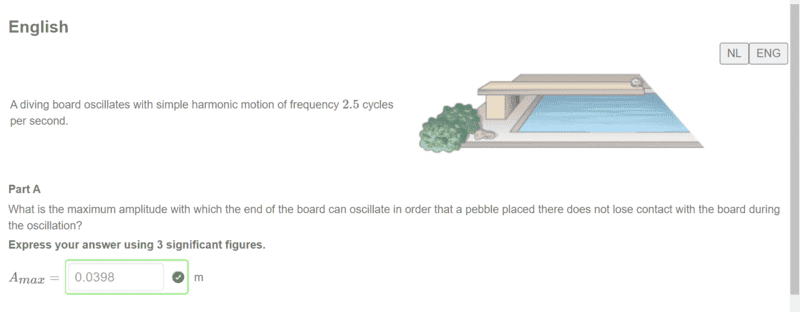How am I supposed to solve this problem?
I did it in a way that's analytically not correct at all lol..
As a first I was thinking about how to relate it to the motion equations to no avail.. (of course with having the condition that normal force board on pebble = force of gravity on pebble)

Then I turned to the fact that the normal force on the board needs to equal the 'spring' force of the board that creates that oscillating motion so...

FBD pebble > ##-mg + N_{board} = 0## > ##N_{board} = mg##
Now.. this is where my analysis is incorrect.
I basically assumed that ##N_{pebble on board} = F_s > mg = kx (1)## where Normal force on board is the same as pebble on board due to nt's 3rd law)
subsituting ##k = \frac {m}{\omega^2}## becomes:
## mg = mx \omega^2##
and then ##x = g \omega^2## with ##\omega = 2 \pi f = 5\pi \frac {rad}{s}##
thus solution: ##x = 0.0398m##

The problem here is that the normal force on the board = spring force has an acceleration , or is that not true.. Meaning.. how should I make an actual correct analysis, because at that point is where I kind of lose the understanding of why I can assume what I assumed I guess.

Edit: also, the acceleration shouldn't be 0 at A_max, so why can we say THA\T ##(1)## is true?

Last edited:
of course with having the condition that normal force board on pebble = force of gravity on pebble
No, not of course. In fact, this is a serious mistake. The entire problem is about finding out when the normal force from the board on the pebble becomes zero.

Think about where in the cycle the pebble would leave the board and why.
The pebble will remain on the board as long as the board does not accelerates downwards faster than the pebble does. When would this happen?

•The spring force will not act on the pebble, the only forces that acts on the pebble is gravity and normal force.

•The spring force will not act on the pebble, the only forces that acts on the pebble is gravity and normal force.
spring force is for the board :)

No, not of course. In fact, this is a serious mistake. The entire problem is about finding out when the normal force from the board on the pebble becomes zero.

Think about where in the cycle the pebble would leave the board and why.
The pebble will remain on the board as long as the board does not accelerates downwards faster than the pebble does. When would this happen?
ooo thank youso this means that there is acceleration which basically becomes 0 and that's where I need to find out at what point it becomes zero, correct?

spring force is for the board :)
it was not clear to me that you have taken that into account.

You basically want to form Newtons second law here, where the forces on the pebble are gravity and normal force, and the acceleration is the same as the acceelration of the board (in the case where it still has contact with the board)

•it was not clear to me that you have taken that into account.

You basically want to form Newtons second law here, where the forces on the pebble are gravity and normal force, and the acceleration is the same as the acceelration of the board (in the case where it still has contact with the board)
oh right...
my whole assumption of acceleration is zero is totally messed up, knew that it shouldn't be correct..

oh right...
my whole assumption of acceleration is zero is totally messed up, knew that it shouldn't be correct..
It is basically the same strategy as you would solve a problem with a car driving on a road that has a circular formed hill, what is the maxium speed for which the car would still have contact with the road. But there you would use centripetal acceleration. In this problem you will use "harmonic oscillation acceleration"

•ooo thank youso this means that there is acceleration which basically becomes 0 and that's where I need to find out at what point it becomes zero, correct?
No. It leaves the board when the downwards acceleration is smaller than that of the board. Since the board acceleration is generally non zero, the required pebble acceleration will be non-zero.

•This picture might help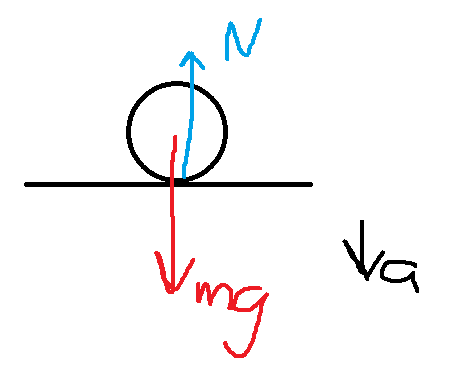•I mean, I wouldn’t even draw a free-body diagram. It is essentially a question of kinematics rather than dynamics.

•It is essentially a question of kinematics rather than dynamics.
Of course it is. But, the contact with the board can be visualized with a free body diagram. Just stating that the acceleration of the board must be greater than the acceleration due to gravity is not providing a direct statement and connection regarding contact or not with the board.

@Orodruin and @malawi_glenn , thanks for the help guys..
I wasn't really sure how to think about this, but it's indeed more of a kinematics probems in an osccillations setting so that confused a bit I guess... For the rest it basically all started with the fact that the normal force is zero at ##A_max##

here is my solution with fbd and stuff as well to see where I get the signs etc
only question I am having... isn't there the force of gravity included for the board as well?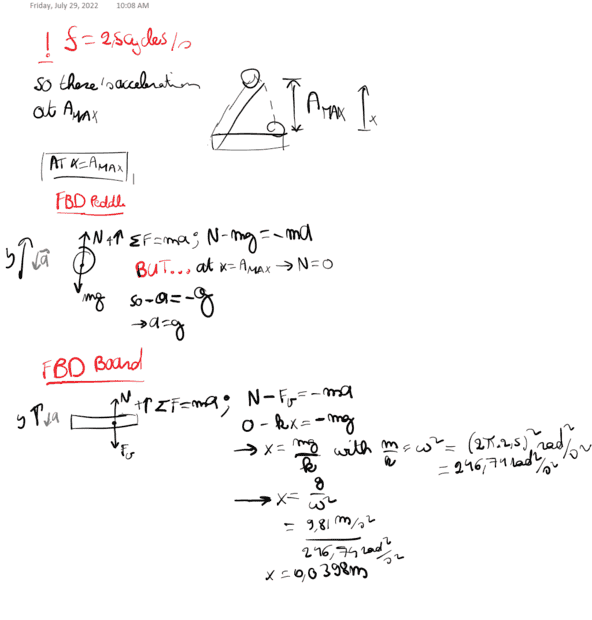•malawi_glenn
Of course it is. But, the contact with the board can be visualized with a free body diagram. Just stating that the acceleration of the board must be greater than the acceleration due to gravity is not providing a direct statement and connection regarding contact or not with the board.
reading this again now .. this totally makes sense to me now (intuitively) as we were talking about the downward acceleration of the plank being bigger at that point.

only problem I am having... isn't there the force of gravity included for the board as well?
The force of gravity on the board is not acting on the pebble, that is the point of FBD. You draw the forces acting on the object of interest.

Just stating that the acceleration of the board must be greater than the acceleration due to gravity is not providing a direct statement
I think a > g is a pretty direct statement…

•I think a > g is a pretty direct statement…
and the normal force on the pebble is 0 then because... (do not mention Newtons 2nd law)

•The force of gravity on the board is not acting on the pebble, that is the point of FBD. You draw the forces acting on the object of interest.
what do you mean? I am sorry, but I don't get what you mean, if we exclude an object at look at its environmental forces, we see the force of gravity aka Earth on board acting, no?
(talking about the second fbd right?)

what do you mean? I am sorry, but I don't get what you mean, if we exclude an object at look at its environmental forces, we see the force of gravity aka Earth on board acting, no?
What is an environmental force?

Are you suggeting that the force of gravitity on the board will influence how the board will oscillate?

You do not need to worry about the board, you only need to know that it oscillates harmonically.

•What is an environmental force?

Are you suggeting that the force of gravitity on the board will influence how the board will oscillate?

You do not need to worry about the board, you only need to know that it oscillates harmonically.
to be more specifice external forces exerted by other objects on the object considered.

so my fbd thing for the board is not correct then or? I am confusedBut to see where I am comming with this I refer to the mass-spring system.
If it is put vertically it's EQ. position will be changed due to the force of gravity

Oh wow.. wait, isn't the plank acting as the 'spring' in that case? right.. and if so than the force of grav of the object on the plank aka the pebble matters.

•hutchphd
What is an environmental force?

Are you suggeting that the force of gravitity on the board will influence how the board will oscillate?

You do not need to worry about the board, you only need to know that it oscillates harmonically.
but okay then why does the gravitiy on the board not influence the board in itself?
or in the cases of a mass spring system the spring itself.

The plank is just like a spring.

I often have this or similar problems on my tests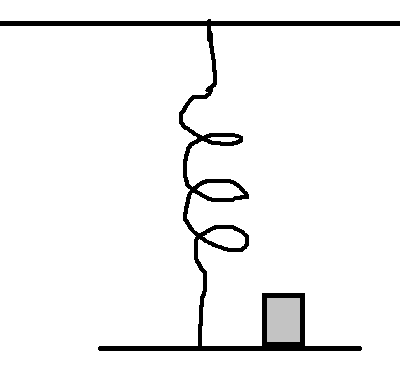given some data regarding the spring, they should figure out the maximal amplitude so that the weight is standing on that platform at all times (sometimes there are 4 identical springs etc)

but okay then why does the gravitiy on the board not influence the board in itself?
You do not need to know anything about the board, except that it oscillates harmonically.

The plank is just like a spring.

I often have this or similar problems on my tests
View attachment 304976
given some data regarding the spring, they should figure out the maximal amplitude so that the weight is standing on that platform at all times (sometimes there are 4 identical springs etc)

You do not need to know anything about the board, except that it oscillates harmonically.
oh yeah indeed similar, that's again normal force = 0
But I guess the thing what I don't understand is.. and right... but the question I am having then is...
How do we relate only that spring force to the acceleration as F_v = ma = mg (both acting downwards)
Because that is what it comes down to and I used the boards fbd which in the aftermath see that that does not make sense now..

How do we relate only that spring force to the acceleration as F_v = ma = mg (both acting downwards)
Do you know how harmonic oscillations work for a vertical hanging spring with a mass attached to it?
It will oscillate around its equilibrium.
There should be a derivation in your textbook.

•Do you know how harmonic oscillations work for a vertical hanging spring with a mass attached to it?
It will oscillate around its equilibrium.
There should be a derivation in your textbook.
That is what I didn't understand at that point. we are looking at

nvm...
I get where I am getting confused... pfff. It is basically the board that is looked at it as the spring and THIS ACTS AS SPRING FORCE ON THE PEBBLE. So my solution is essentially wrong and the spring force needs to be added to the pebble ain't that right?

ACTS AS SPRING FORCE ON THE PEBBLE. So my solution is essentially wrong and the spring force needs to be added to the pebble ain't that right?
No it doesent.
The forces acting on the pebble is gravity and normal force.

•You only need to know what the pebbles acceleration is, which is this case is acceleration due to harmonic oscillation. As long as the pebble has contact with the board, it will undergo same acceleration as the board.

Last edited:
•You do not need to know anything about the "spring force" acting on the board, you only need to know that its motion is that of an harmoic oscillator.
to be more correct hooke's law is what I meant..
oehhh that is tough haha, if it were the first time it'd get such a question I would most definitely use the mass of platform as well lol.

But I am sorry, but it's still not getting through.
like hooke's law gives us the restoring force.
Normal force on the plank due to pebble. So we only relate those because these contribute to the oscillation?
And thereby we ignore the force of gravity of the plank.

The FBD you drew for the plank is not nessecary, and it is wrong, because the board is attached to something at its left end.

And thereby we ignore the force of gravity of the plank.
No, forget about the plank. You only need the forces acting on the pebble and what the acceleration of the pebble is, which is the same as the acceleration of the board when the pebble has contact with it. And since you know that the board is undergoing harmonic oscillation and you have its frequency, you are done.

I would most definitely use the mass of platform as well lol.
Well it depends on what is given in the problem of course, but I always give - similar to this problem - the frequency or the period or something.

•The FBD you drew for the plank is not nessecary, and it is wrong, because the board is attached to something at its left end.

No, forget about the plank. You only need the forces acting on the pebble and what the acceleration of the pebble is, which is the same as the acceleration of the board when the pebble has contact with it. And since you know that the board is undergoing harmonic oscillation and you have its frequency, you are done.
right, so how do I relate the result for the acceleration to the hooke's law, if we have a normal force and the force of gravity (let me write it out real quick)

I sorry but I just don't get the translation from a mass-spring used in the textbook to the real world application where it's not attached.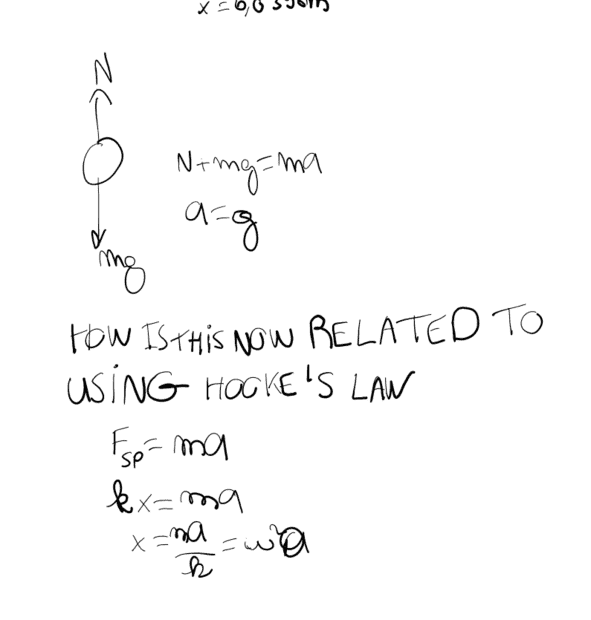here it is (to add is it by knowing that we have harmonic osciallation we could simply apply this to situation of no mass-spring system acting in accordance to hooke's law or what?)

here it is
yeah, in magnitude that is.
This is a general property of acceleration due to harmonic motion, the magnitude of the acceleration is proportional to the magnitude displacement. The constant of proportionality is the angular velocity squared, and the direction of the acceleration is oppsoite to the displacement.
You can see it here as well, the position as a funtion of time is sinusodial ##x(t) = A \sin (\omega t + \varphi)## The acceleration is the second derivative w.r.t. time. By differenting twice, we get ##a(t) = -\omega ^2 x(t)## and the maximal acceleration is therefore (in magnitude) ##\omega^2 A##

•yeah, in magnitude that is.
This is a general property of acceleration due to harmonic motion, the magnitude of the acceleration is proportional to the magnitude displacement. The constant of proportionality is the angular velocity squared, and the direction of the acceleration is oppsoite to the displacement.
You can see it here as well, the position as a funtion of time is sinusodial ##x(t) = A \sin (\omega t + \varphi)## The acceleration is the second derivative w.r.t. time. By differenting twice, we get ##a(t) = -\omega ^2 x(t)## and the maximal acceleration is therefore (in magnitude) ##\omega^2 A##
oh I am so dumb... it is just ##a_{max} = \omega^2 A_{max}##
The analysis of what I did will just show me what the ACCELERATION IS FROM THE KINETICS/DYNAMICS PART OF VIEW
BUT THEN GOING BACK TO THE KINEMATICS PART WE HAVE MOTION ACCORDING TO THE HARMONIC EQUATIONS, correct?

•malawi_glenn
Thanks for the help... I don't see where I was getting all of this from..
Because the acceleration essentially goes by ##a = A\omega^2 cos(\omega t + \phi)## and has nothing to do with the relation ##F_s = ma (kx = mg)##

I guess I was trying too much to look at it as a mass-spring system and relate it to the board case which not the way to go (talking about incorrectly looking at the dynamics part of the mass spring system and relating that to the board-peddle system), but just a case of oscillation

•malawi_glenn